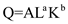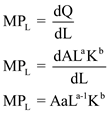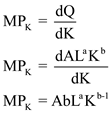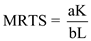# Quiz 9: Production and Cost in the Long Run

Business

In the long-run the advice will prove beneficial for BlueStar. This is because, in the long-run there is an opportunity for Blue Star to add more aircraft with same passenger load. The same passenger load implies that the quantity Q remains constant in the long run. The constant quantity will help BlueStar to reduce the average cost and the addition made to the average cost is less than that made to the total cost. Thus, with a decline in the long-run average cost, the average total cost also decreases. Economies of scale refer to the reduction of costs due to increase in size, output, or scale operations. If the firm expands the output by introducing the new plant, this will decrease the total cost of the production. This means, the industry will experience the economies scale by adding aircraft to the fleet. Thus, the addition of more aircrafts in the fleet will reduce the long run average cost and BlueStar will get to experience the economies of scale.

The following is the production function of a firm:(A) The marginal product of labor (MP L ) is derived by differentiating the production function with respect to labor (L):(B) The marginal product of labor (MP K ) is derived by differentiating the production function with respect to capital (K):(C) Marginal rate of technical substitution (MRTS) is the rate at which one input is substituted for other.(D) Isoquants are convex if MRTS is decreasing for increasing units of labor:For increasing labor units in denominator, the MRTS falls. Thus MRTS is a diminishing function and hence convex. (E) Along the expansion path, the slope of the cost curve (W/r) is equal to the marginal rate of technical substitution (MRTS):The ratio of marginal product from an input and price of input determines the productivity of that input. - The marginal product of printer ( MP PT ) is 20 and wage rate is \$20. - The marginal product of printing press ( MP PR ) is 1000 and its price is \$5000.- The marginal product and price ratio is higher for printer than printing press. Thus, the company should replace printer instead of printing press.

There is no answer for this question